﻿ 防城港进出港船舶交通组织研究

# 防城港进出港船舶交通组织研究Study on the Traffic Management of Ships Entering and Leaving the Fangcheng Port

Abstract: To ensure the normal operation of the traffic organization during ships entering and leaving port, a channel passing capacity estimation model is established based on the time-space consumption theory. The model considers the time resources and space resources (area) of the channel as the total resources of the channel. The passing capacity and service level of each channel of Fangcheng Port are obtained by calculation. The methods of combining cellular automata and multi-agents are used to establish a simulation model of Fangcheng Port’s navigable capacity. Simulation experiments show that the Fangcheng Port channel can meet the requirements of ships entering and leaving the port.

1. 引言

2. 航道通过能力计算模型建立

2.1. 基于时空消耗理论的航道通过能力计算模型

${C}_{channel}^{b}=\frac{R}{{R}_{ship}}=\frac{AT}{{R}_{ship}}$ (1)

${C}_{channel}^{d}={R}_{1}×{R}_{2}×{R}_{3}×{R}_{4}×{R}_{5}×{R}_{6}×{R}_{7}×{C}_{channel}^{b}$ (2)

1) 航道空间资源计算方法如下式所示。

${A}_{channel}={L}_{channel}×W×N$ (3)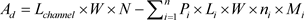(4)

2) 航道时间资源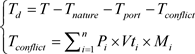(5)

3) 单船消耗的航道总资源

$\begin{array}{c}{R}_{ship}=\left({L}_{distance}+{L}_{ship}\right)×W×{T}_{ship}\\ =\left({L}_{distance}+{L}_{ship}\right)×W×\frac{{L}_{channel}}{V}\end{array}$ (6)

4) 航道通过能力计算模型(7)

2.2. 船舶调度对航道通过能力的影响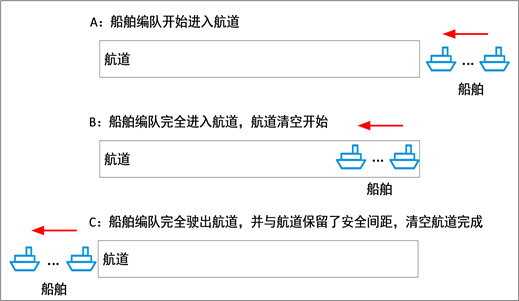Figure 1. Schematic diagram of channel clearing process

${t}_{clean}=\frac{{L}_{channel}+{L}_{distance}}{V}$ (8)

${T}_{port}=\left(k-1\right)×{t}_{clean}=\left(k-1\right)×\frac{{L}_{channel}+{L}_{distance}}{V}$ (9)

$\left\{\begin{array}{l}{T}_{in-port}=m×{t}_{clean-in}+{T}_{out}=m×\frac{{L}_{channel}+{L}_{distance}}{{V}_{in}}+{T}_{out}\\ {T}_{out-port}=n×{t}_{clean-out}+{T}_{in}=n×\frac{{L}_{channel}+{L}_{distance}}{{V}_{out}}+{T}_{in}\end{array}$ (10)

$饱和度=\frac{Q}{C}$ (11)Table 1. Standards for dividing waterway operation levels

2.3. 航道通过能力计算Table 2. Ship traffic flow ship length, speed distribution

2.3.1. 现阶段通过能力

1) 实际船舶流量计算

2) 航道通过能力计算Table 3. Calculation results of passing capacity at this stage

2.3.2. 近期通过能力Table 4. Result of recent passing capacity calculationTable 5. Channel passing ability under different dispatching rules

2.3.3. 中期通过能力Table 6. Calculation results of mid-term passing capacity under different scheduling rules

2.3.4. 远期通过能力Table 7. Long-term passing capacity calculation result

3. 仿真验证与分析

3.1. 防城港通过能力仿真模型构建

3.1.1. 建模方法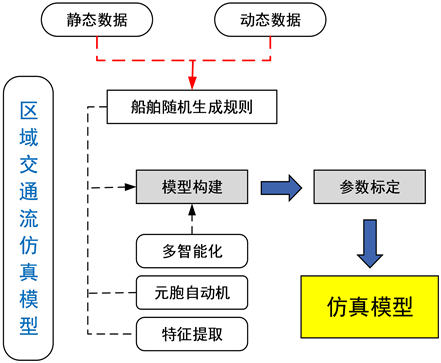Figure 2. Model structure

3.1.2. 模型实现

1) 根据元胞自动机的规则，将航道进行离散化，并确定航道边界；

2) 将时间进行离散化为可被主程序识别的模块；

3) 根据各目标水域航道、定线制的具体情况，构建对应的航道多智能体模块；

4) 根据泊位的地理位置、尺度信息等，构建泊位多智能体模型。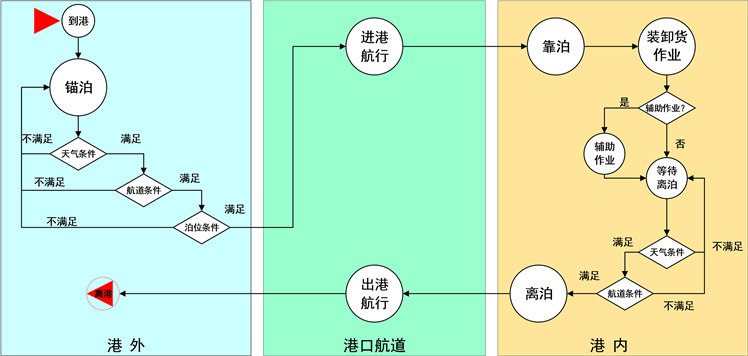Figure 3. Simulation system logic model

$P\left(t\right)=1-{\text{e}}^{-t/\lambda }$

${T}_{k+1}={T}_{k}+R$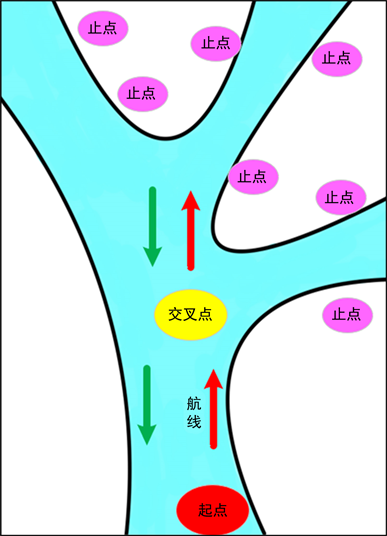Figure 4. Airway model

1) 到港船舶能否加入进港队列进港，需满足条件：

${D}_{S-{S}_{in}^{n}}\ge {D}_{saf}$

2) 船舶能汇出，需满足条件：

${D}_{s}^{out}\ge {D}_{saf}^{cross}$

${D}_{s}^{out}$ 表示汇出船舶拟穿越的出港队列中的两船之间的距离； ${D}_{saf}^{cross}$ 为允许穿越的最小安全距离。

3) 船舶能否汇入，需满足条件：

${D}_{os}^{in}\ge {D}_{saf}^{cross}$，且 ${D}_{os}^{out}\ge {D}_{saf}^{in}$

${D}_{os}^{in}$ 表示汇入船舶拟穿越的进港队列中的两船之间的距离； ${D}_{os}^{out}$ 表示汇入船舶拟汇入的出港队列中的两船之间的距离； ${D}_{saf}^{in}$ 表示允许汇入的最小安全距离。

3.2. 基于仿真方法的目标水域通过能力分析

3.2.1. 仿真工况Table 8. Distribution parameters of berth service time for ships of different lengths

1) 在锚地生成船舶。

2) 由于航道的差异，船舶在不同航道中的通航能力是不同的，当任何一段航道达到饱和，即为港口船舶服务能力。

3) 锚地生成船舶的数量不断增加，直到船舶不能按照管理规定实现船舶进出港作业。

4) 满足任意一段航道的通航能力，船舶锚泊所需锚地，被定义为所需要锚地。

3.2.2. 仿真结果Table 9. Entry and exit conditions under different restrictions

4. 结论

 Uğurlu, Ö., Yüksekyıldız, E. and Köse, E. (2014) Simulation Model on Determining of Port Capacity and Queue Size: A Case Study for BOTAS Ceyhan Marine Terminal. The International Journal on Marine Navigation and Safety of Sea Transportation, 8, 143-150.

 丁涛, 徐湘文. 基于OD结构网络模型的长江干线航道通过能力研究[J]. 武汉理工大学学报(交通科学与工程版), 2015, 39(4): 751-754.

 朱俊, 张玮. 基于跟驰理论的内河航道通过能力计算模型[J]. 交通运输工程学报, 2009, 9(5): 83-87.

 Kumaran, S.K., Dogra, D.P. and Roy, P.P. (2019) Queuing Theory Guided Intelligent Traffic Scheduling through Video Analysis Using Dirichlet Process Mixture Model. Expert Systems with Applications, 118, 169-181.
https://doi.org/10.1016/j.eswa.2018.09.057

 李文杰, 龙浩, 戴佳伶, 杨胜发, 杨威, 张先炳. 基于排队理论的单线控制河段航道通过能力研究[J]. 水运工程, 2020(1): 97-102.

 Dragovic, B., Tzannatos, E. and Park, N. (2017) Simulation Modelling in Ports and Container Terminals: Literature Overview and Analysis by Research Field, Application Area and Tool. Flexible Services & Manufacturing Journal, 29, 4-34.
https://doi.org/10.1007/s10696-016-9239-5

 王更, 许才广. 基于Flexsim的深圳港铜鼓航道通过能力仿真研究[J]. 水运工程, 2019(9): 181-185+195.

 孟宁, 陈燕, 王军. 多浅段航道设计通过能力仿真[J]. 大连海事大学学报, 2011, 37(1): 63-67+86.

 戴林伟. 双向航道船舶交通流元胞自动机模型及仿真[J]. 上海海事大学学报, 2019, 40(1): 27-31+64.

Top